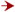# The XOR Problem and Solution

An architectural Solution to the XOR Problem

Now here's a problem. Let's try to build a neural network that will produce the following truth table, called the 'exclusive or' or 'XOR' (either A or B but not both):

a b a XOR b
1 1 0
0 1 1
1 0 1
0 0 0

In order to solve the problem, we need to introduce a new layer into our neural networks. This layer, often called the 'hidden layer', allows the network to create and maintain internal representations of the input. Here's is a network with a hidden layer that will produce the XOR truth table above:

XOR Network

From Rumelhart, et al. 1986, p 64.

Fair enough. Recall that one of the strengths of neural networks that makes them different from symbolic networks is that the rules for the manipulation of the input symbols are 'learned', not pre-loaded into the system. This solution relies on a certain network architecture, and that architecture is pre-defined, just like the rules of a symbolic system. The challenge, then, for neural network simulations is to create a system that can change its architecture depending on the outputs that the network creates.

The idea is simple: we set the network up so that it takes in inputs and produces an output. If the output produced does not match the output we desired, the network will create an 'error signal', and pass that error signal backwards through the network to the input nodes. As the error is passed, we change the network architecture so that the error is minimized. So how might we do it?

First, we allow each connection to have a weight that is not just '1' or '-1'. Second, we drop the 'threshold' for each node, and add a 'bias'. A node's bias is simply a constant that is added to the total input to determine that node's activation. Third, in the last network architecture, a node became active if and only if the net input was greater than the threshold. Now that we no longer have a threshold, we have to change the function that activates the network. We change the rule for activation to the following:Where 'Net Input' is the sum of all the inputs to the given node. The input is, remember, the product of the activation of the node sending the input and the weight of the connection.

Now, in order to make the network reorganize its architecture, we present the network with various inputs and the output desired for that input. In the case of the XOR problem, those inputs and outputs are set by the truth table:

a b a XOR b
1 1 0
0 1 1
1 0 1
0 0 0

The training proceeds in five stages. First, we create the network with random weights and random biases. Second, we set the activation of the two input nodes from the columns 'a' and 'b' in the table, and run the network forward. Third, we compare the output produced by the network to the desired output (in the third column of the truth table), and calculate the difference between the actual output and the desired output. This difference is the error signal. Fourth, we change the weights of the connections that connect to the output node, and the bias of the output node. Fifth, we pass the error back to the hidden layer, and change the biases and weights of those connections. Then the cycle repeats with new inputs and new outputs. The network trains until the average error (calculated over all four rows in the truth table) approaches zero.

Press 'Run' to begin the network. Once the network is created and the weights and biases randomized, press 'train'. You will see the connection weights and node biases change as the network trains. Once the network has completed its training phase, set the inputs to '1' or '0', and press 'Step' to see the output. The output node will show it's activation.

Back Propogation Solution

Because of the nature of the activation function, the activity on the output node can never reach either '0' or '1'. We take values of less than 0.1 as equal to 0, and greater than 0.9 as equal to 1.

If the network seems to be stuck, it has hit what is called a 'local minimum'. Keep your eye on the bias of the hidden node and wait. It will eventually head towards zero. As it approaches zero, the network will get out of the local minimum, and will shortly complete. This is because of a 'momentum turn' that is used in the calculation of the weights.

In the last module, created a network that would simulate an 'Inverse conditional'. Here's the truth table for a material conditional:

a b ab
1 1 1
0 1 0
1 0 1
0 0 1

Backpropagation is able to solve this problem easily. More importantly, we don't need to change the structure of the program, or the architecture of the network, all we have to do is change the target truth table:

Conditional Backpropagation Network

In fact, this network can learn any logical relationship expressible in a truth table of this sort. In the following, you can change the desired output, and train the network to produce that output.

Backpropagation for Any Binary Logical Function